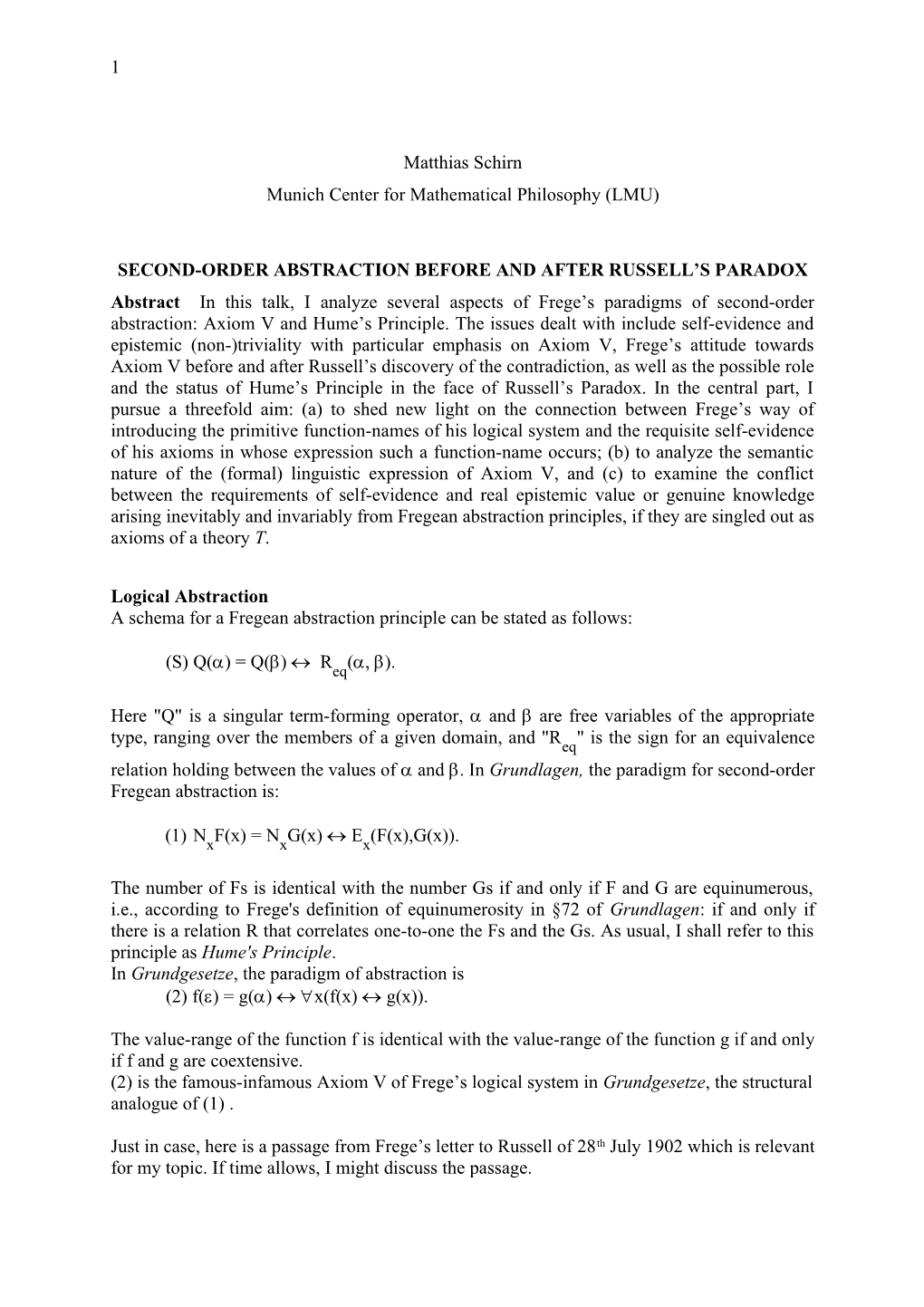# Second-Order Abstraction Before and After Russell S Paradox1

Matthias Schirn

Munich Center for Mathematical Philosophy (LMU)

SECOND-ORDER ABSTRACTION BEFORE AND AFTER RUSSELL’S PARADOX

Abstract In this talk, I analyze several aspects of Frege’s paradigms of second-order abstraction: Axiom V and Hume’s Principle. The issues dealt with include self-evidence and epistemic (non-)triviality with particular emphasis on Axiom V, Frege’s attitude towards Axiom V before and after Russell’s discovery of the contradiction, as well as the possible role and the status of Hume’s Principle in the face of Russell’s Paradox. In the central part, I pursue a threefold aim: (a) to shed new light on the connection between Frege’s way of introducing the primitive function-names of his logical system and the requisite self-evidence of his axioms in whose expression such a function-name occurs; (b) to analyze the semantic nature of the (formal) linguistic expression of Axiom V, and (c) to examine the conflict between the requirements of self-evidence and real epistemic value or genuine knowledge arising inevitably and invariably from Fregean abstraction principles, if they are singled out as axioms of a theory T.

Logical Abstraction

A schema for a Fregean abstraction principle can be stated as follows:

(S) Q() = Q()  Req(, ).

Here "Q" is a singular term-forming operator,  and  are free variables of the appropriate type, ranging over the members of a given domain, and "Req" is the sign for an equivalence relation holding between the values of  and . In Grundlagen, the paradigm for second-order Fregean abstraction is:

(1)NxF(x) = NxG(x) Ex(F(x),G(x)).

The number of Fs is identical with the number Gs if and only if F and G are equinumerous, i.e., according to Frege's definition of equinumerosity in §72 of Grundlagen: if and only if there is a relation R that correlates one-to-one the Fs and the Gs. As usual, I shall refer to this principle as Hume's Principle.

In Grundgesetze, the paradigm of abstraction is

(2) f() = g() x(f(x) g(x)).

The value-range of the function f is identical with the value-range of the function g if and only if f and g are coextensive.

(2) is the famous-infamous Axiom V of Frege’s logical system in Grundgesetze,the structural analogue of (1) .

Just in case, here is apassage from Frege’s letter to Russell of 28th July 1902 which is relevant for my topic. If time allows, I might discuss the passage.

I myself was long reluctant to acknowledge value-ranges and hence classes; but I saw no other possibility of placing arithmetic on a logical foundation. But the question is: How do we grasp logical objects? And I have found no other answer to it than this: We grasp them as extensions of concepts, or more generally, as value-ranges of functions. I have always been aware that there are difficulties connected with this, and your discovery of the contradiction has added to them; but what other way is there?... We can also try to help ourselves in the following way [Man kann sich auch so zu helfen suchen] and I hinted at this in my Grundlagen der Arithmetik. If we have a relation ) for which the following sentences hold: 1. from a,b) follows b, a); 2. from a, b) and b, c) follows a,c), then this relation can be transformed into an equality (identity), and a, b) can be replaced by saying, e.g., §a = §b. If the relation is that of geometrical similarity, then for “a is similar to b” we can say “the shape of a is the same as the shape of b”. This is perhaps what you call “définition par abstraction”. But the difficulties here are the same as in the transformation of a generality of an equality into a value-range equality.

The choice of an abstraction principle as an axiom of a theory T: Frege’s dilemma

The epistemic dilemma that I shall discuss applies especially to Axiom V, but it would equally apply to Hume’s Principle, if Frege were to select it as an axiom of a theory T, or to the choice of any other abstraction principle. Here is some preparatory information:

I assume that according to Frege (a) the epistemic triviality of a thought goes hand in hand with its self-evidence, while (b) the converse does not hold generally. An axiom must be self-evident, but at the same time it should have real epistemic value or contain genuine knowledge. The foundation of a fruitful axiomatic theory cannot consist of sheer trivialities. (c) If in an equation of the form “a = b” the expressions “a” and “b” not only refer to the same object, but also express the same sense, then “a = b” is epistemically trivial.“a = b” can be converted into an equation of the form “a = a” without any change of sense; and such an equation is undoubtedly trivial. It is, moreover, true by virtue of its form and therefore a truth of logic. This is of course not to say that any logical truth is trivial which, according to Frege, is clearly not the case. (d) An equation of the form “a = b” to which (c) applies, is, according to (a), self-evident. (e) The self-evidence of a true equation of the form “a = b” implies that “a” and “b” express the same sense and, hence, according to (c) that “a = b” is epistemically trivial. (f) If in a true equation of the form “a = b” “a” and “b” express different senses, then “a = b” is neither self-evident nor epistemically trivial.